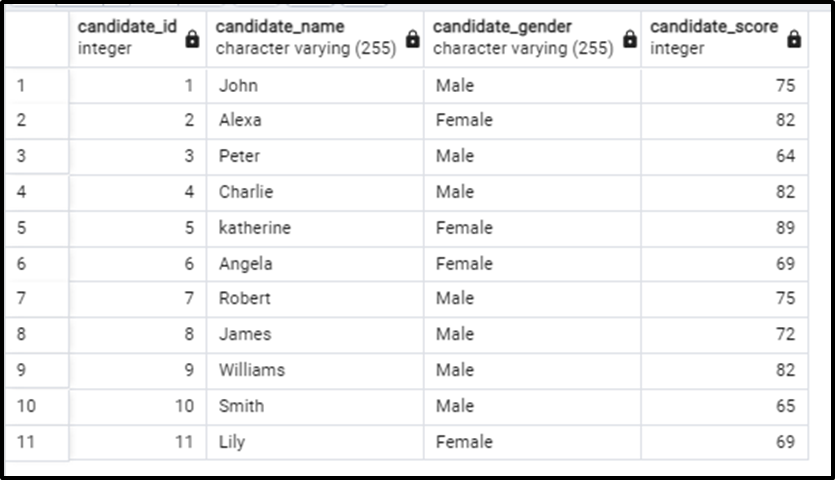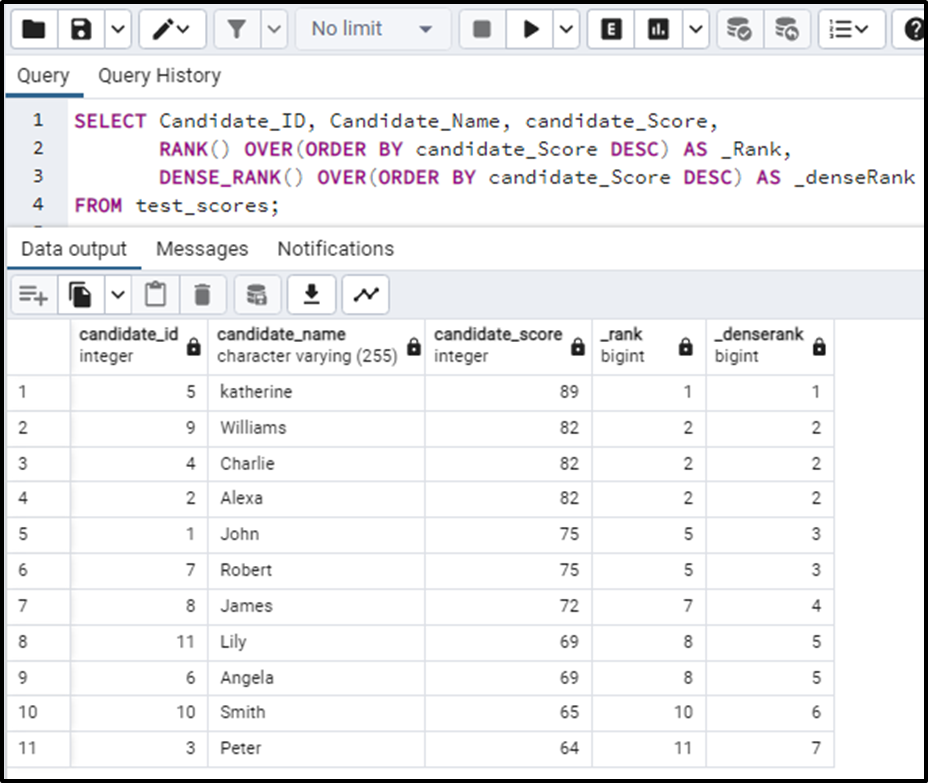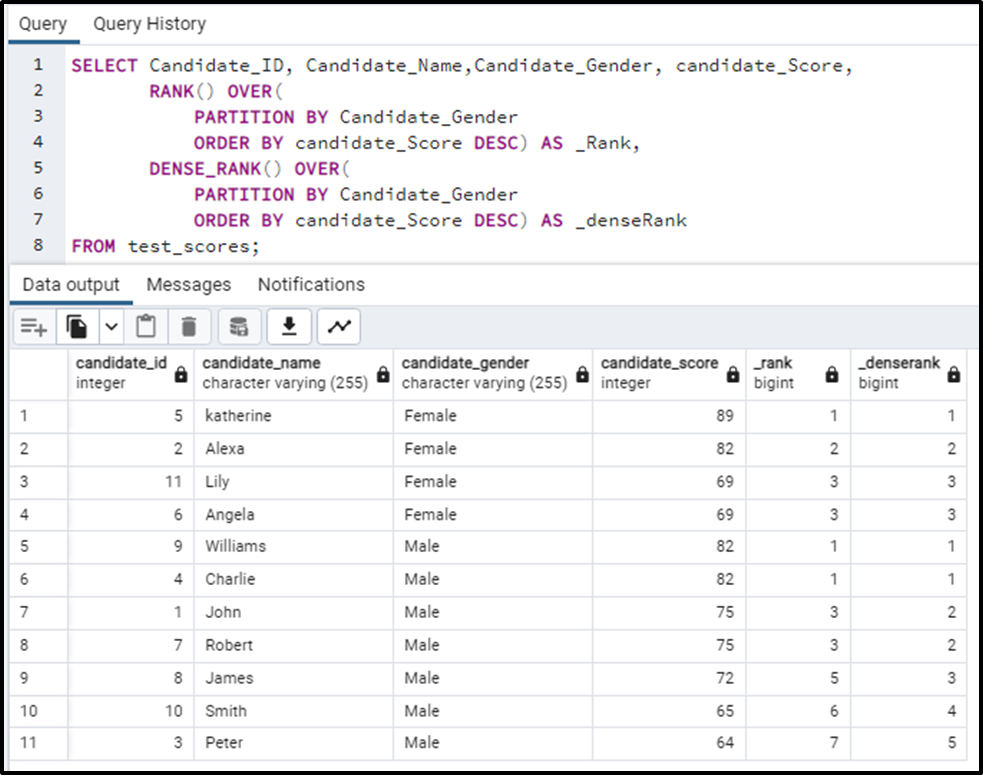##### RANK() versus DENSE_RANK() in Postgres: What is the Difference?

PostgreSQL provides built-in functions that aid in assigning rankings to table rows. Among these functions, the most commonly and frequently used functions are RANK() and DENSE_RANK(). These functions assign sequential ranks, with both functions assigning an equal rank to identical data elements. The difference in approach comes after assigning the same ranks to the elements.

Let's discuss the details of both functions and see how they are different.

RANK() Vs DENSE_RANK() in Postgres: What is the Difference?

RANK() and DENSE_RANK() functions are ranking functions. They both rank the data according to order and assign the same rank to the same elements in the table. Let's see how they both particularly work.

RANK()

The RANK() function allocates those elements with the same rank, which are identical. The rank of the next element will be the current value of rank plus the number of times the previous element repeated.

The syntax for the RANK() function is illustrated below:

`RANK() OVER ( [PARTITION BY partition_expression, ... ] ORDER BY sort_expression [ASC | DESC], ... )`

DENSE_RANK()

DENSE_RANK() function gives the same rank to those elements which are the same. The rank of the next element will be the next consecutive value to the previous rank.

The basic syntax of DENSE_RANK() function is:

`DENSE_RANK() OVER ([PARTITION BY partition_expression, ... ] ORDER BY sort_expression [ASC | DESC], ... )`

Let’s discuss the examples so that the syntax and the differences in both are much clearer.

Example 1: RANK() Vs DENSE_RANK() in Postgres

Consider the table showing the scores of the candidates appearing in an entry test of a university. The table contains columns for id, name, gender, and score.Now we will apply the RANK() and DENSE_RANK() functions to the above-given table. The query will be written as follows:

```SELECT Candidate_ID, Candidate_Name, candidate_Score,
RANK() OVER(ORDER BY candidate_Score DESC) AS _Rank,
DENSE_RANK() OVER(ORDER BY candidate_Score DESC) AS _denseRank
FROM test_scores;```

In the above code:

● The SELECT statement is followed by the column names that are to be selected from the test_scores table.

● ORDER BY clause in both cases is necessary in order to sort our data. This sorting can be in ascending or descending order. We can decide the type of sorting as per our use case requirements. Here in our case, this order needs to be in descending order so that the candidate with the highest marks gets the first rank and so on. Here we ordered the column candidate_Score in descending order.

● Lastly, we have applied the RANK() and DENSE_RANK() functions on the ordered data and stored the rank in the columns: _Rank and _denseRank in the table respectively.

The output of the above query is:In the above output, we can clearly see the difference between the two functions. Let's analyze both one by one.

In the case of the RANK() function, every(same) element is assigned the same rank. But the pattern to be noticed is the very next rank after the same rank assignment. When rank gives the same rank to one or many elements the next element’s rank is calculated as: The current value of rank plus number of times the previous element repeated.

For example in the table given above, the score 89 is a single element so the rank assigned to it is 1. The next value is 82, all the 82’s will be assigned rank 2. Here notice the 82 is repeated 3 times. So the rank of the next element will be calculated as:The rank of the next element, that is, 75 is 5. And 75 is again repeated 2 times so the rank of the next element 72 is 7(i.e. 5+2). 72 occurs once so the rank for the next element (69) is 8. 69 is again duplicated so the rank for both of the 69s is 8 and the rank for the next element is 10(i.e. 8+2). The last two elements occur once so both will be assigned rank 10 and 11 respectively.

In the case of the DENSE_RANK() function, every (same) element is assigned the same rank. The rank assigned to the next element will be the next consecutive value to the rank. This is simple. Just analyze the example. The dense rank for 89 will be 1. The dense rank for the next element(82) will be 2. 82 occurs 3 times so all 82s will be given rank 2 but this thing will not affect the rank of the next element. The rank for the next element will still be the consecutive rank i.e. 3. And this way the dense rank works.

Example 2: Comparing RANK() and DENSE_RANK() Functions With PARTITION BY in Postgres

Another important thing that we can do is rank the data by partitioning it under some conditions. For example; in the above-considered example, we can partition the data based on gender and then rank it. This thing can be done if we use the PARTITION BY clause with these ranking functions. This clause is completely optional as we have seen in the above example, however, if the data is not partitioned using the PARTITIONED BY clause these queries will consider the whole data as one partition. Let's see how it works.

The above query is written as:

```SELECT Candidate_ID, Candidate_Name,Candidate_Gender, candidate_Score,
RANK() OVER(
PARTITION BY Candidate_Gender
ORDER BY candidate_Score DESC) AS _Rank,
DENSE_RANK() OVER(
PARTITION BY Candidate_Gender
ORDER BY candidate_Score DESC) AS _denseRank
FROM test_scores;```

You will see the clear partitioning based on gender and will observe the ranking patterns in the output of the above query:The ranking functions have ranked the data according to the partitions formed based on gender.

Conclusion:

Rank() and DENSE_RANK() are the functions used to rank the data. Both functions have some functionality in common and some differences are also there. We can use the PARTITION BY clause with these functions and this clause is a completely optional clause. It partitions the data into parts on the basis of some attribute and then ranks these segments using ranking functions according to their respective partitions. If the PARTITION BY clause is not present, these functions consider the data as one partition by default.